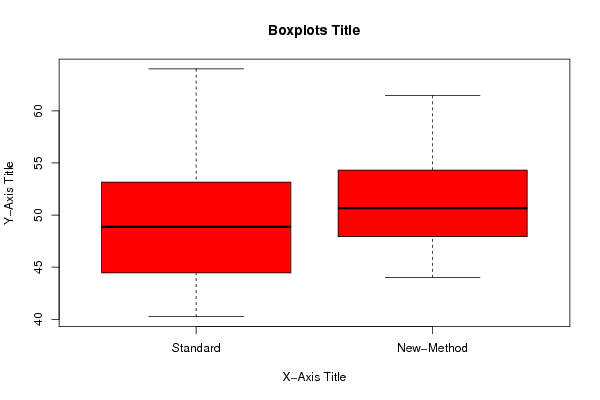## Free Statistics

of Irreproducible Research!

Author's title
Author*The author of this computation has been verified*
R Software Modulerwasp_Tests to Compare Two Means.wasp
Title produced by softwareT-Tests
Date of computationFri, 11 Nov 2011 10:22:06 -0500
Cite this page as followsStatistical Computations at FreeStatistics.org, Office for Research Development and Education, URL https://freestatistics.org/blog/index.php?v=date/2011/Nov/11/t13210249377inp2ajt8657zq7.htm/, Retrieved Wed, 04 Oct 2023 01:10:01 +0000
Statistical Computations at FreeStatistics.org, Office for Research Development and Education, URL https://freestatistics.org/blog/index.php?pk=141361, Retrieved Wed, 04 Oct 2023 01:10:01 +0000
QR Codes:Original text written by user:
IsPrivate?No (this computation is public)
User-defined keywords
Estimated Impact120
Family? (F = Feedback message, R = changed R code, M = changed R Module, P = changed Parameters, D = changed Data)
-     [Aston University Statistical Software] [Morning Sickness ...] [2009-11-16 16:26:06] [74be16979710d4c4e7c6647856088456]
- R     [Aston University Statistical Software] [Morning Sickness ...] [2009-11-16 17:22:16] [74be16979710d4c4e7c6647856088456]
-   P     [T-Tests] [Morning Sickness ...] [2010-11-09 11:12:43] [3fdd735c61ad38cbc9b3393dc997cdb7]
- RM        [T-Tests] [Morning Sickness ...] [2011-11-07 09:34:35] [98fd0e87c3eb04e0cc2efde01dbafab6]
- R           [T-Tests] [] [2011-11-10 13:35:02] [84f9d24ffbb976b91a97c3ec996667ce]
-    D            [T-Tests] [] [2011-11-11 15:22:06] [eefd59af29ef05ead55189a23e75ec4c] [Current]
Feedback Forum

Post a new message
Dataseries X:
41.81	46.12
40.25	50.9
50.37	47.32
43.12	47.05
40.46	49.55
42.89	48.33
54.29	44.49
56.13	51.64
51.76	46.55
42.92	54.79
45.58	47.51
47.37	50.83
48.27	48.41
47.02	53.83
52.23	52.95
64.02	56.88
52.36	48.5
53.97	53.29
60.45	54.06
47.76	56.66
47.44	48.88
48.35	44.46
49.21	49.63
52.12	49.12
44.39	56.88
48.89	46.89
54.04	55.97
40.31	50.42
50.51	52.67
52.33	51.28
44.52	55.89
55.59	54.55
54.27	48.8
49.18	52.39
40.75	56.8
45.36	46.69
43.54	44.01
48.82	50.51
54.05	56.01
57.47	61.45

 Summary of computational transaction Raw Input view raw input (R code) Raw Output view raw output of R engine Computing time 1 seconds R Server 'Gwilym Jenkins' @ jenkins.wessa.net

\begin{tabular}{lllllllll}
\hline
Summary of computational transaction \tabularnewline
Raw Input & view raw input (R code)  \tabularnewline
Raw Output & view raw output of R engine  \tabularnewline
Computing time & 1 seconds \tabularnewline
R Server & 'Gwilym Jenkins' @ jenkins.wessa.net \tabularnewline
\hline
\end{tabular}
%Source: https://freestatistics.org/blog/index.php?pk=141361&T=0

[TABLE]
[ROW][C]Summary of computational transaction[/C][/ROW]
[ROW][C]Raw Input[/C][C]view raw input (R code) [/C][/ROW]
[ROW][C]Raw Output[/C][C]view raw output of R engine [/C][/ROW]
[ROW][C]Computing time[/C][C]1 seconds[/C][/ROW]
[ROW][C]R Server[/C][C]'Gwilym Jenkins' @ jenkins.wessa.net[/C][/ROW]
[/TABLE]
Source: https://freestatistics.org/blog/index.php?pk=141361&T=0

Globally Unique Identifier (entire table): ba.freestatistics.org/blog/index.php?pk=141361&T=0

As an alternative you can also use a QR Code:

The GUIDs for individual cells are displayed in the table below:

 Summary of computational transaction Raw Input view raw input (R code) Raw Output view raw output of R engine Computing time 1 seconds R Server 'Gwilym Jenkins' @ jenkins.wessa.net

 T-Test Mean1 49.10425 Mean2 51.074 T Statistic -1.76752 P-value 0.08143 Lower Confidence Limit -4.19175 Upper Confidence Limit 0.25225

\begin{tabular}{lllllllll}
\hline
T-Test \tabularnewline
Mean1 & 49.10425 \tabularnewline
Mean2 & 51.074 \tabularnewline
T Statistic & -1.76752 \tabularnewline
P-value & 0.08143 \tabularnewline
Lower Confidence Limit & -4.19175 \tabularnewline
Upper Confidence Limit & 0.25225 \tabularnewline
\hline
\end{tabular}
%Source: https://freestatistics.org/blog/index.php?pk=141361&T=1

[TABLE]
[ROW][C]T-Test[/C][/ROW]
[ROW][C]Mean1[/C][C]49.10425[/C][/ROW]
[ROW][C]Mean2[/C][C]51.074[/C][/ROW]
[ROW][C]T Statistic[/C][C]-1.76752[/C][/ROW]
[ROW][C]P-value[/C][C]0.08143[/C][/ROW]
[ROW][C]Lower Confidence Limit[/C][C]-4.19175[/C][/ROW]
[ROW][C]Upper Confidence Limit[/C][C]0.25225[/C][/ROW]
[/TABLE]
Source: https://freestatistics.org/blog/index.php?pk=141361&T=1

Globally Unique Identifier (entire table): ba.freestatistics.org/blog/index.php?pk=141361&T=1

As an alternative you can also use a QR Code:

The GUIDs for individual cells are displayed in the table below:

 T-Test Mean1 49.10425 Mean2 51.074 T Statistic -1.76752 P-value 0.08143 Lower Confidence Limit -4.19175 Upper Confidence Limit 0.25225

 Standard Deviations Variable 1 5.70493 Variable 2 4.1389

\begin{tabular}{lllllllll}
\hline
Standard Deviations \tabularnewline
Variable 1 & 5.70493 \tabularnewline
Variable 2 & 4.1389 \tabularnewline
\hline
\end{tabular}
%Source: https://freestatistics.org/blog/index.php?pk=141361&T=2

[TABLE]
[ROW][C]Standard Deviations[/C][/ROW]
[ROW][C]Variable 1[/C][C]5.70493[/C][/ROW]
[ROW][C]Variable 2[/C][C]4.1389[/C][/ROW]
[/TABLE]
Source: https://freestatistics.org/blog/index.php?pk=141361&T=2

Globally Unique Identifier (entire table): ba.freestatistics.org/blog/index.php?pk=141361&T=2

As an alternative you can also use a QR Code:

The GUIDs for individual cells are displayed in the table below:

 Standard Deviations Variable 1 5.70493 Variable 2 4.1389PNG link Postscript link PDF link

Parameters (Session):
par1 = two.sided ; par2 = 1 ; par3 = 2 ; par4 = T-Test ; par5 = unpaired ; par6 = 0.0 ; par7 = 0.95 ; par8 = TRUE ;
Parameters (R input):
par1 = two.sided ; par2 = 1 ; par3 = 2 ; par4 = T-Test ; par5 = unpaired ; par6 = 0.0 ; par7 = 0.95 ; par8 = TRUE ;
R code (references can be found in the software module):
par2 <- as.numeric(par2)par3 <- as.numeric(par3)par4 <- as.character(par4)par5 <- as.character(par5)par6 <- as.numeric(par6)par7 <- as.numeric(par7)par8 <- as.logical(par8)if ( par5 == 'unpaired') paired <- FALSE else paired <- TRUEx <- t(y)if(par8){bitmap(file='test1.png')(r<-boxplot(x ,xlab=xlab,ylab=ylab,main=main,notch=FALSE,col=2))dev.off()}load(file='createtable')if( par4 == 'Wilcoxon-Mann_Whitney'){a<-table.start()a <- table.row.start(a)a <- table.element(a,'Wilcoxon Test',3,TRUE)a <- table.row.end(a)a <- table.row.start(a)a <- table.element(a,'',1,TRUE)a <- table.element(a,'Statistic',1,TRUE)a <- table.element(a,'P-value',1,TRUE)a <- table.row.end(a)W <- wilcox.test(x[,par2],x[,par3],alternative=par1, paired = paired)a<-table.row.start(a)a<-table.element(a,'Wilcoxon Test',1,TRUE)a<-table.element(a,W$statistic[])a<-table.element(a,round(W$p.value, digits=5) )a<-table.row.end(a)a<-table.end(a)table.save(a,file='mytable.tab')}if( par4 == 'T-Test'){T <- t.test(x[,par2],x[,par3],alternative=par1, paired=paired, mu=par6, conf.level=par7)a<-table.start()a <- table.row.start(a)a <- table.element(a,'T-Test',3,TRUE)a <- table.row.end(a)if(paired){a <- table.row.start(a)a <- table.element(a,'Difference: Mean1 - Mean2',1,TRUE)a<-table.element(a,round(T$estimate, digits=5) )a <- table.row.end(a)}if(!paired){a <- table.row.start(a)a <- table.element(a,'Mean1',1,TRUE)a<-table.element(a,round(T$estimate, digits=5) )a <- table.row.end(a)a <- table.row.start(a)a <- table.element(a,'Mean2',1,TRUE)a<-table.element(a,round(T$estimate, digits=5) )a <- table.row.end(a)}a <- table.row.start(a)a <- table.element(a,'T Statistic',1,TRUE)a<-table.element(a,round(T$statistic, digits=5) )a <- table.row.end(a)a <- table.row.start(a)a <- table.element(a,'P-value',1,TRUE)a<-table.element(a,round(T$p.value, digits=5) )a <- table.row.end(a)a <- table.row.start(a)a <- table.element(a,'Lower Confidence Limit',1,TRUE)a<-table.element(a,round(T$conf.int, digits=5) )a <- table.row.end(a)a<-table.row.start(a)a <- table.element(a,'Upper Confidence Limit',1,TRUE)a<-table.element(a,round(T\$conf.int, digits=5) )a <- table.row.end(a)a<-table.end(a)table.save(a,file='mytable.tab')}a<-table.start()a <- table.row.start(a)a <- table.element(a,'Standard Deviations',3,TRUE)a <- table.row.end(a)a <- table.row.start(a)a <- table.element(a,'Variable 1',1,TRUE)a<-table.element(a,round(sd(x[,par2]), digits=5) )a <- table.row.end(a)a <- table.row.start(a)a <- table.element(a,'Variable 2',1,TRUE)a<-table.element(a,round(sd(x[,par3]), digits=5) )a <- table.row.end(a)a<-table.end(a)table.save(a,file='mytable1.tab')